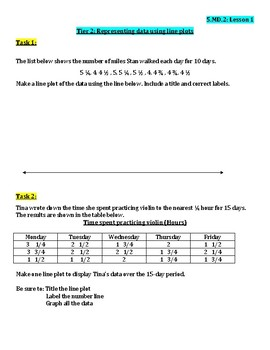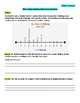# Grade 5 Unit 4 Measurement & Geometry Tiered Work BundleSubject
Resource Type
File Type
PDF (1 MB|65 pages)
\$48.00
Product Description
Tiered Work is a great way to differentiate for the day's math lesson plan. Use the Exit Slips to guide which tier students should start their independent work with.

0/2 questions correct=See teacher
1/2 questions correct=Tier 1 (computation)
2/2 questions correct=Tier 2 (two-step word problems)
Move to Tier 3 after completing Tier 1 and/or Tier 2 (multi-step word problems)

Unit 4 Measurement and Geometry
-Lesson 1 Line plots representing data
-Lesson 2 Interpret data using a line plot
-Lesson 3 Understand the concept of volume
-Lesson 4 Counting unit cubes volume
-Lesson 5 Use a formula to determine the volume
-Lesson 6 Find the volume of a composite figure
-Lesson 7 Convert customary units of length
-Lesson 8 Convert customary capacity
-Lesson 9 Convert customary weight
-Lesson 10 Convert metric length
-Lesson 11 Convert metric capacity
-Lesson 12 Convert metric weight
-Lesson 13 Convert units of time
Total Pages
65 pages